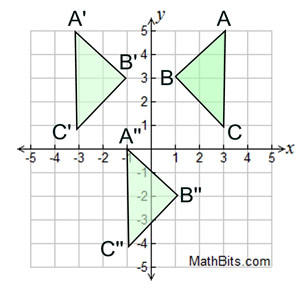Review Practice - Grid Transformations Terms of Use    Contact Person: Donna Roberts1.
Which of the following choices describes the transformation of ΔABC shown in the graph at the right?

Choose:
 reflection over the x-axis reflection over the y-axis rotation of 90º counterclockwise        (centered at the origin) rotation of 90º clockwise        (centered at the origin)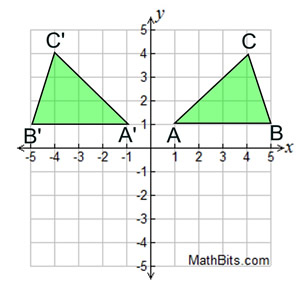2.
Triangle CAT has vertices C (2,3), A(-2,6) and T(-1,-4). A translation maps the point A to A' (-1,4). What are the coordinates of C' under this translation?

Choose:
 (1,7) (1,1) (3,1) (3,5)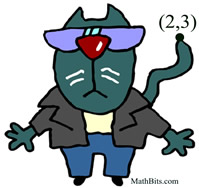3.
Which of the following choices describes the transformation of TRAP, as shown in the graph at the right?

Choose:
 (x, y) → (x + 3, y + 5) (x, y) → (x - 3, y - 5) (x, y) → (x + 3, y - 5) (x, y) → (x - 3, y + 5)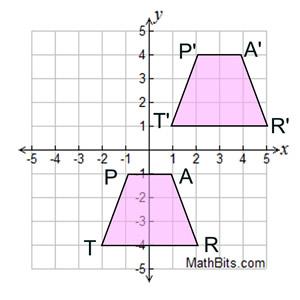4.
The shape shown in the diagram at the right is reflected over the x-axis and then reflected over the y-axis.
a) What are the coordinates of the image of point P after these two transformations?
Choose:
 (4,3) (3,-4) (-4,3) (-3,-4)

b)
Which choice represents the orientation of the shape after the two transformations?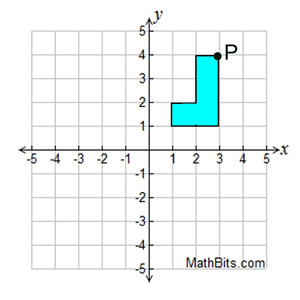Choose: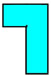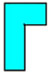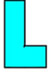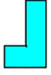5.
ΔABC, with A(4,5), B(-2,0), and C(0,-4), is to be reflected over the x-axis. Which of the vertices, if any, will remain static (will not change its position) during the transformation?
Choose:
 A B C All of the vertices will change.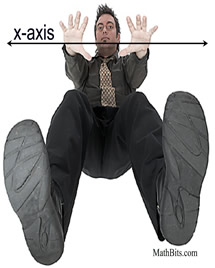6.
ΔABC and its reflection can be seen in the graph at the right. What is the equation of the line of reflection?

Choose:
 y = 0 y = -1 y = 1 y = -3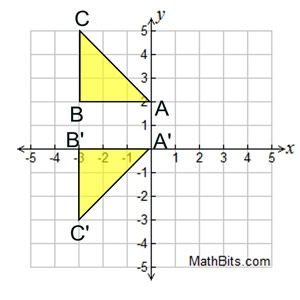7.
What single translation accomplishes the same as the translation
(x, y) → (x + 1, y - 2)
followed by
(x, y) → (x - 4, y + 5)
followed by
(x, y) → (x - 3, y - 1)?
Choose:
 (x, y) → (x + 8, y + 8) (x, y) → (x + 6, y - 2) (x, y) → (x - 6, y + 2) (x, y) → (x - 8, y - 8)8.
Triangle ABC is to be transformed under a dilation in the origin with a scale factor of 1/3.
What are the coordinates of the image of vertex C under this dilation?

Choose:
 (0,1) (1,-1/3) (-1,-1) (-9,-9)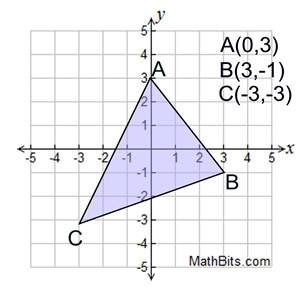9.
A dilation in the origin with a scale factor of 2 is applied to ΔABC.
What is the length of A'B' ?

Choose:
 6 units 8 units 12 units 18 units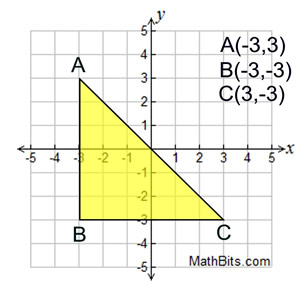10.
Which of the following descriptions (pertaining to the graph at the right) is true?
Choose:
 ΔA"B"C" is a translation of ΔABC. ΔA"B"C" is a translation of ΔA'B'C'. ΔA"B"C" is a dilation in the origin of scale factor 2 of ΔABC. ΔA'B'C' is a translation of ΔABC.